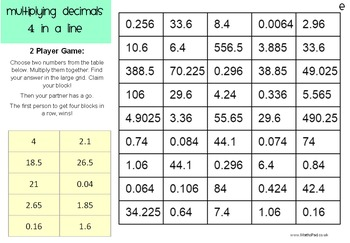# Multiplying Decimals: Four in a Line GamesSubject
Resource Type
Common Core Standards
Product Rating
File Type

PDF (Acrobat) Document File

1 MB|6 pages
Share
Product Description
This pack contains six different Four in a Line Games based around multiplying decimals:

Games 1 & 2: Multiplying and dividing decimals by 10, 100 and 1000.
Students take it in turns to choose a number from a set provided and can multiply or divide it by 10, 100 or 1000 to make a number on the board. The first person to make four numbers in a line wins!

Games 3 & 4: Easier Multiplying Decimals
Students have to multiply two decimals together to make a number on the board. These games include fairly simple calculations like 0.7 x 6

Games 5 & 6: Harder Multiplying Decimals
In these games students will have to multiply numbers like 24.6 and 1.4 in order to make a number on the board and win the game!

Like this? Check out my pre-algebra bundle here to save \$\$!

Common Core: 5.NBT.B.7
Add, subtract, multiply, and divide decimals
Total Pages
6 pages